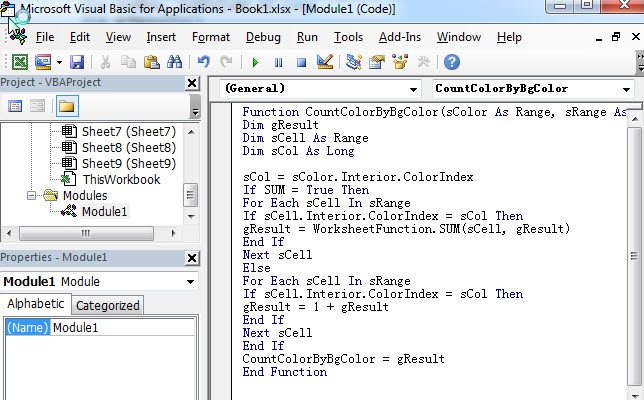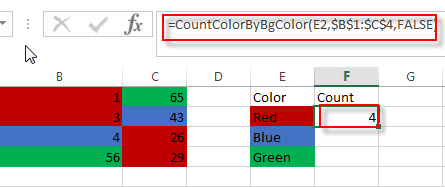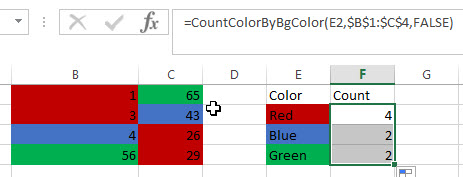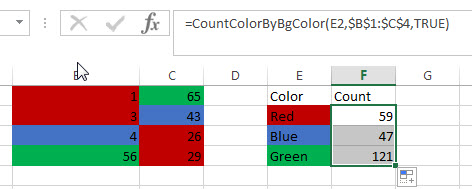# How to count and sum cells by background color

This post will guide you how to count and sum cell by background color in excel. How to get the sum of colored cells in the current worksheet. How do I count cells by background color of cells with user defined function in excel VBA. How to Sum cells by background color in excel.

## Count and sum cells by background color

There is no particular function which use to count or sum cells by color in Microsoft excel, so you can try to write a new user defined function to sum or count cells based on background color.

Suppose you have a table where the range of cells in the current worksheet are colored. And if you want to count the number of colored cells, such as, the number of blue or red color in this range of cells, you can create a new user defined function to reflect this requirement.

So you can create the below user defined function to count and sum cells by background color of cells in Excel VBA, just refer to the below steps:

1# open your excel workbook and then click on “Visual Basic” command under DEVELOPER Tab, or just press “ALT+F11” shortcut.2# then the “Visual Basic Editor” window will appear.

3# click “Insert” ->”Module” to create a new module4# paste the below VBA code into the code window. Then clicking “Save” button.

```Function CountColorByBgColor(sColor As Range, sRange As Range, Optional SUM As Boolean)
Dim gResult
Dim sCell As Range
Dim sCol As Long

sCol = sColor.Interior.ColorIndex
If SUM = True Then
For Each sCell In sRange
If sCell.Interior.ColorIndex = sCol Then
gResult = WorksheetFunction.SUM(sCell, gResult)
End If
Next sCell
Else
For Each sCell In sRange
If sCell.Interior.ColorIndex = sCol Then
gResult = 1 + gResult
End If
Next sCell
End If
CountColorByBgColor = gResult
End Function```5# back to the current worksheet, try to enter the below formula to count cells by background color in Cell F2:

`=CountColorByBgColor(E2,\$B\$1:\$C\$4,FALSE)`You can see that the number of red color of cells in range B1:C4 has been calculated to Cell F2.

If you want to count the number of others color in the range B1:C4, you just need to drag AutoFill handle down to other cells.If you want to sum the cells by background color in the range B1:C4, you can write down the following formula using the above user defined functions:

`=CountColorByBgColor(E2,\$B\$1:\$C\$4,TRUE)`So you just need to set the third argument as TRUE, and it will do SUM action to sum colored cells.